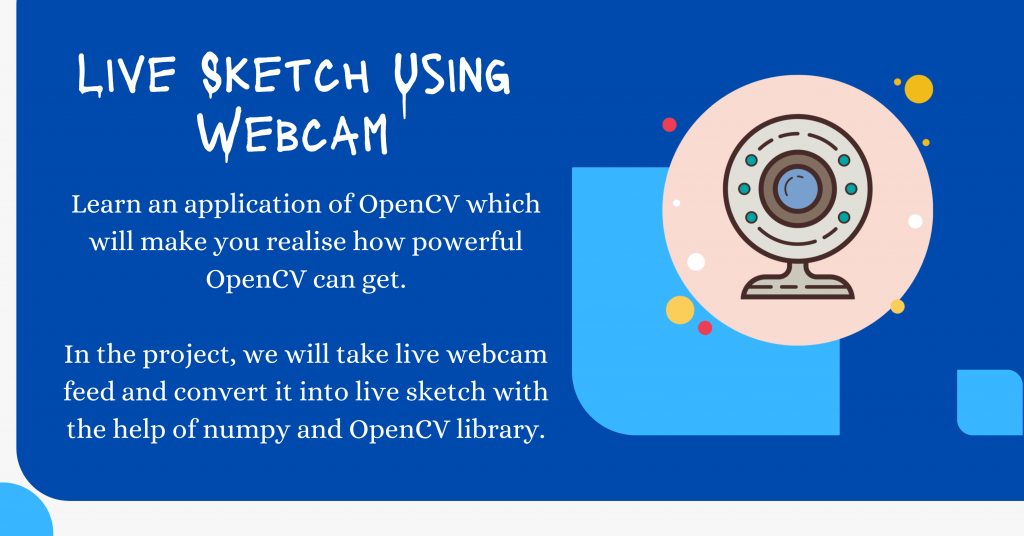# Live Sketch Using Webcam with Python OpenCV [Easy Guide]Today in this tutorial, you will learn an application of OpenCV which will make you realize how powerful OpenCV can get.

In the project, we will take a live webcam feed and convert it into a live sketch with the help of numpy and OpenCV library.

Let’s begin with the amazing project!

## Step 1: Importing Modules

To get started, we need to import `OpenCV` and `Numpy` (assuming that you have the libraries installed). We define OpenCV and Numpy in our code as follows:

```import cv2
import numpy as np
```

## Step 2: Define a function to convert frame to Sketch

In order to covert a frame into a sketch we will follow a number of steps which are listed below:

1. Convert Image into a `gray` image
2. Apply `Gaussian Blur` to the gray image obtained
3. Apply `Canny Edge Detection` to the Gaussian Image
4. In the end, Invert the Image and get the `Binary Inverted Image`

The code for the function is shown below.

```def sketch(image):
img_gray = cv2.cvtColor(image, cv2.COLOR_BGR2GRAY)
img_gray_blur = cv2.GaussianBlur(img_gray, (5,5), 0)
canny_edges = cv2.Canny(img_gray_blur, 10, 70)
ret, mask = cv2.threshold(canny_edges, 70, 255, cv2.THRESH_BINARY_INV)
```

## Step 3: Open Live Webcam and apply function

We need to use the webcam and extract image frames from the video. In order to achieve the same, we will use the `VideoCapture` and `read` function to extract frames one after another.

Now use the `imshow` function to display the live webcam and apply the sketch function created in the previous step.

The final step is to create an exit condition for the window. Here we have kept the key the `Enter Key` as the exit key for the window. In the end, destroy all the windows that were opened and yet to be closed during the program.

```cap = cv2.VideoCapture(0)
while True:
cv2.imshow('Our Live Sketcher', sketch(frame))
if cv2.waitKey(1) == 13: #13 is the Enter Key
break
cap.release()
cv2.destroyAllWindows()
```

## The Complete Code

```import cv2
import numpy as np

def sketch(image):
img_gray = cv2.cvtColor(image, cv2.COLOR_BGR2GRAY)
img_gray_blur = cv2.GaussianBlur(img_gray, (5,5), 0)
canny_edges = cv2.Canny(img_gray_blur, 10, 70)
ret, mask = cv2.threshold(canny_edges, 70, 255, cv2.THRESH_BINARY_INV)

cap = cv2.VideoCapture(0)
while True:
cv2.imshow('Our Live Sketcher', sketch(frame))
if cv2.waitKey(1) == 13: #13 is the Enter Key
break
cap.release()
cv2.destroyAllWindows()
```

## The Final Output

The small video below displays the final output obtained after running the whole code mentioned in the previous section.

## Conclusion

I hope you understood the concept and loved the output. Try out the simple code yourself and observe the power of the OpenCV library.

Happy Coding! 😇